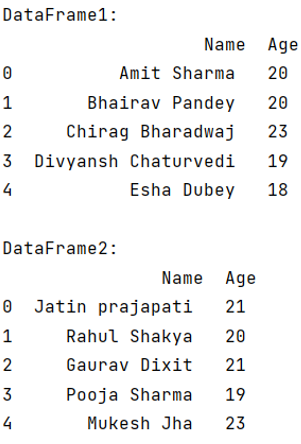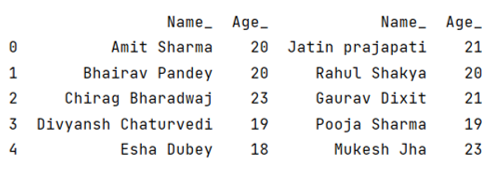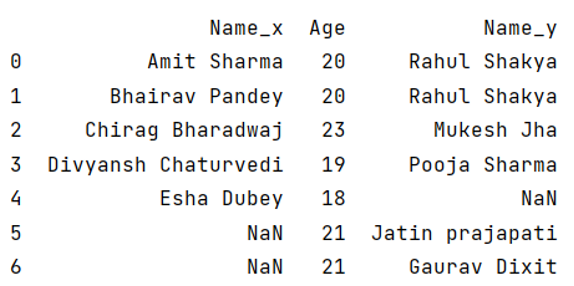# What is the difference between join and merge in Pandas?

Learn about the main differences between join and merge in Python Pandas. By Pranit Sharma Last updated : September 20, 2023

Pandas is a special tool which allows us to perform complex manipulations of data effectively and efficiently. Inside pandas, we mostly deal with a dataset in the form of DataFrame. DataFrames are 2-dimensional data structure in pandas. DataFrames consists of rows, columns and the data.

Pandas provides numerous ways to combine two Series or DataFames in order to perform effective and efficient data analytics. Sometimes our required data is not present in a single DataFrame and in that case we need to combine two or more DataFrames.

## Difference between join() and merge() methods in Pandas

Pandas merge() and pandas join() are both the methods of combining or joining two DataFrames but the key difference between is that join() method allows us to combine the DataFrames on the basis of the index i.e., the row value, whereas the merge() method allows us to combine the DataFrames on the basis of specific columns instead of index values.

## Example to demonstrate the difference between join() and merge() methods

For better understanding, let us first create a DataFrame,

```# Importing pandas package
import pandas as pd

# Creating a Dictionary
dict1 = {
'Name':['Amit Sharma','Bhairav Pandey','Chirag Bharadwaj','Divyansh Chaturvedi','Esha Dubey'],
'Age':[20,20,23,19,18]
}

dict2 = {
'Name':['Jatin Prajapati','Rahul Shakya','Gaurav Dixit','Pooja Sharma','Mukesh Jha'],
'Age':[21,20,21,19,23]
}

# Creating a DataFrame
df1 = pd.DataFrame(dict1)
df2 = pd.DataFrame(dict2)

# Display DataFrame
print("DataFrame1:\n",df1,"\n")
print("DataFrame2:\n",df2,"\n")
```

Output:Now we will apply merge() and join() separately on these DataFrames to understand the functional difference.

## join() Method

```# Using join method
df_join = df1.join(df2, lsuffix='_')

# Display method
print(df_join)
```

Output:Here, the join method combines the two DataFrames on the basis of their indexes, and we can observe from the above example, that the second DataFrame is simply added to the first DataFrame with properly aligned rows. Also, since our column names are same for both the DataFrames, we have assigned a left suffix to the first DataFrame to distinguish the two DataFrames and to prevent from overlapping.

## merge() Method

```# Using merge method
df_merged = df1.merge(df2, on='Age', how='outer')

# Display result
print(df_merged)
```

Output: